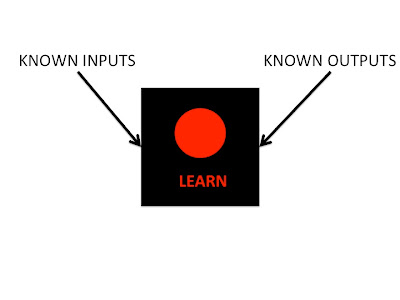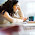## Monday, April 2, 2012

### How Fish Shoal (4): Using a Neural Network to Learn From Data

To recap, in the first post on this topic, I started by asking how we can use recorded data of fish movements in groups to learn how they interact. I stated that we can see this as inferring a function between the environment and the fish's behaviour, and in the subsequent posts we looked at how we might estimate functions using regression, arriving at the idea of a neural network as a highly flexible tool for performing non-linear regression. In this post we'll see how we can practically use neural networks (as one possible tool among many alternatives) to learn from data, and how this is actually coded in Matlab to show how few of the details we need to concern ourselves with to start doing useful inference.

Inference always begins by deciding which outputs (behaviours) we want to predict from which inputs (stimuli, environment). The recorded positions of the various fish over time taken from video tracking are only useful once we make this assignment. In the case of our research we looked at the relative positions and directions of each fish's neighbours in the group as the inputs, and the fish's responses of accelerations (or deceleration) and turning angle, as shown in the figure below taken from our paper.The relative position and direction of a neighbour (yellow) from the focal fish (red)
So first we take all the recorded positions of the fish, and for every fish at every time step we calculate the following quantities:

1. The angle (theta) and distance (r) to the nearest neighbour, the second nearest neighbour, third nearest etc.

2. The direction (phi) of each neighbour relative to the focal fish

3. How much the fish accelerated (a) and turned (alpha) on the next time step

We also measure quantities associated with where the wall of the tank is relative to the fish, but I'll ignore these for now. 1 and 2 here are our inputs, the stimuli. 3 is the behaviours - what the fish did next in response to the stimuli.

So, assuming that we've tracked our fish and measured the above, lets get inferring....

Let's try seeing how the acceleration of the focal fish is related to the position of the nearest neighbour. Once you've got Netlab installed, you can build a neural network in just one line

my_nn = mlp(2, 10, 1, 'linear');

my_nn: is your neural network (remember from the last post, it can also be called a Multi-Layer Peceptron - mlp)

2: is the number of inputs we want to use. We will be using the angle and distance to the neighbour.

10: is the number of 'hidden nodes' - thats the number of nodes in the middle layer of the diagram we saw in the last post. We can change this number - more nodes make the network more flexible but harder to learn well. I find 20 tends to work ok, but always experiment! Each node will be a sigmoidal function of the inputs, but we're not going to worry about these details here.

'linear': means that the output will be a weighted sum of all the hidden nodes. The only real reason to change this is if the outputs are binary rather than continuous.

Now you have a neural network! But at the moment is doesn't do very much. It's been configured in a random state. You can try putting some numbers in and seeing what come out using mlpfwd

y = mlpfwd(my_nn, [x1, x2]);

where x1and x2 are any possible values of the angle and distance to the nearest fish you want to try, and y is the predicted acceleration. At the moment those predictions will be meaningless, as the network hasn't learnt anything.

Now comes the useful bit. Assume we have three vectors containing the data, theta is a vector of angles to the nearest fish, r is a vector of the distances to the nearest fish and a is a vector of how much the fish accelerated. Make sure these are column vectors. Then we can train network using just a few more lines of code.

options = zeros(1, 18); options(1)=1; options(14) = 100;
my_nn = netopt(my_nn, options, [theta, r], a, 'scg');

netopt is a function that trains the network, based on the data its given. It tries to find the values for all the parameters (like the 'slopes' in the last post) which will produce the best match between what actually comes out of the network when we put the inputs (position of the nearest neighbour) in, and the behaviours we tell it should come out (i.e. the measured accelerations). options is, as the name suggests a number of possible options. Here we only use 2. The first tells Matlab to show the error values as the algorithm learns, the 14th tells netopt to run 100 iterations of the learning algorithm. The learning algorithm is something called 'scaled conjugate gradients', which is the 'scg' at the end.

Now we can input any values of theta and r to the network and it should output a value of the expected acceleration that fits with the data it has already seen. That is about 90% of everything you need to know to start doing inference with a neural network today. All the diagrams and equations in the last post are nice to have in the back of your head while doing this, but essentially you can treat the neural network as a black box. You put data in, in the form of known inputs and outputs. You press a button to make the network 'learn', and then the box will tell you what output you should expect for any input you offer it.First we show the network some known examples..then we ask it to predict the output for other inputs
This is in fact the basis of pretty much all of machine-learning. Take a number of known examples of something, such as images of handwritten letters. Plug them into a learning algorithm (of which a neural network is but one among many) to train it. Then use the same algorithm to predict what some unknown examples are.

Now all that remains is to try inputting all the possible values of theta and r that we might be interested in. In our paper we made the further simplification that the function would be symmetric around the axis of the fish - i.e. if the fish will accelerate when a neighbour is ahead on the left, it will also do so if the neighbour is ahead on the right. So we test values of r between 0 and some maximum (like 40cm), and angles between 0 and pi (everywhere on the left of the fish). In Matlab we can make vectors of these test inputs like this:

r_test = linspace(0, 40, 100);
theta_test = linspace(0, pi, 100); %this gives us 100 values of each input

[r_grid, theta_grid] = ndgrid(r_test, theta_test);
test_input = [r_grid(:), theta_grid(:)];
%this matches every value of r to every value of theta so we can test all pairs

test_acc = mlpfwd(my_nn, test_input); %this puts our test inputs through the network we learned

test_acc = reshape(test_acc, size(r_grid));
%and this makes the output accelerations into a matrix so we can visualise it

X = cos(theta_test)*r_test';
Y = sin(theta_test)*r_test';
pcolor(X, Y, test_acc);
%This visualises the output on a nice semi-circle

And so finally we get a plot showing what the network thinks the fish will do for any given position of the nearest neighbouring fishThat B is because this comes from a multipanel image, as we'll see soon
So we confirm some previously held beliefs about how interactions like this work. The focal fish accelerates to catch up with a neighbour in front. It slows down to rejoin a neighbour behind. And if a neighbour is too close (near the centre), this is reversed to move the focal fish to a more comfortable distance.

So in a few lines of code by us, and a lot of preprogrammed code by the makers of Netlab, we have done some quite sophisticated inference with a minimum of real maths. Of course, there are some complications in now getting from the 90% you already know to the 100% you need to get publication ready. You'll need to concern yourself things like multiple local minima of the squared error, cross-validation and such other things. But these are things to worry about once you've got your hands a little dirty and started actually doing some inference...none of them mean you can't start applying these techniques to your data TODAY!

In the next and probably last post on this topic I'll show how we go from learning this relatively simple function with just 2 inputs, to a more complex function accounting for the positions of many neighbours, and we'll investigate the perils of correlation and confounding.

[Again, if you want to read more about the details of any of these techniques, I recommend David Mackay's textbook, Information Theory, Inference and Learning Algorithms (free online). Netlab also contains a large number of demo scripts, of which demomlp1.m demo is similar to this post]

#### 1 comment:

1.